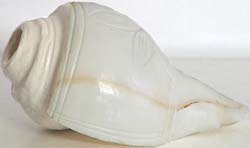# Sri Lanka Numerology.

There are plenty Astrologist cum Numerologist in Sri Lanka. Currently Mrs. Easwari and Molivanan are very popular in Colombo for numerology calculated under the Sri Lankan method. Numbers do play a big role in the life of human. They can give you good, bad and mixed fortunes in your life.

There are numbers from 1 - 9 and 0 is not counted in side of the numerology as it doesn't have any value as it is empty. Also if you are looking for the answer for who invented the numerology ? We are 100% sure it was not inventedby Sri Lankans. Sri Lanka was introduced with the English alphabets by the then ruled Europeans. So we can assume this kind of astrology is not our ancient people's one. Although Tamil people knew about the value of numbers and they respected some numbers like 9, 108 and 1008 as had values for worshiping the god. 9 is very important as it represents 9 openings of the human body. Because of this the Hindu temples are made resembling the human body with 9 gates to it and the Kopuram too built with 9 windows. Most of the temple festivals take place for 9 days and flowers, pots and conch shells were took in to pooja ceremonies in 108 and 1008.

Planet Sun rules 1, 10, 19 and 28

Planet Moon rules 2, 11, 20 and 29.

Planet Jupiter rules 3, 12, 21 and 30.

Planet Rahu rules 4, 13, 22 and 31.

Planet Mercury rules 5, 14 and 23.

Planet Venus rules 6, 15 and 24.

Planet Ketu rules 7, 16 and 25.

Planet Saturn rules 8, 17 and 26.

Planet Mars rules 9, 18 and 27.

1 is the power for the letters A I JQ Y

2 is the power for the letters B C K R

3 is the power for the letters G L S

4 is the power for the letters D M T

5 is the power for the letters: N E

6 is the power for the letters for the letters U V W X

7 is the power for the letters for the letters O Z

8 is the power for the letters F H P

9 No alphabet is allocated for this number in the Sri Lanka numerology.

A=1

B=2

C=3

D=4

E=5

F=8

G=3

H=5

I=1

J=1

K=2

L=3

M=4

N=5

O=7

P=8

Q=1

R=2

S=3

T=4

U=6

V=6

W=6

X=5

Y=1

Z=7

If your Name is M.Sooriyanarayanan, here is the way how to calculte your name's numerological value.

M.Sooriyanarayanan

4+ 3 + 7 + 7 + 2 + 1 + 1 + 1 + 5 + 1 + 2 + 1 + 1 + 1 + 5 + 1 + 5 = 48 = 4 + 8 = 12 = 1 + 2 = 3

You are under the Jupiter Planet's control.